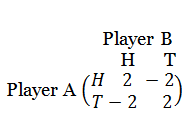# Game Theory Fundamentals

Posted by   on

Game Theory:
A game theory consists of
- A list of perticipants
- A specification of choices open to each other.
- Specification of the way in which the choices determine result realized by each player.

Characteristics of  a game:
1. The number of players is finite.
2. There is a conflict of interest among players.
3. Each player has a finite number of choices for possible courses of action referred to as strategies.
4. Rules governing the choice of actions are known to each other.
5. The outcome of the game is affected by the choices made by the competitor
and
6. All combination of courses of action chosen by various players always result in some outcome of the game denoting gain/loss of an individual player. This outcome can be represented by a single pay-off number that can be zero (no gain, no loss), positive (gain) and negative (loss).

Payoff:
The amount mi received by the player Pi from other participant is called the payoff of the player Pi.
This is the outcome of the game.
A payoff matrix is the table (matrix) which shows the amounts received by the  player named at the left hand side after all possible plays of the game.
The payment is made by the player at the top of the table.

Zero sum game:
If the players make payments only for each other i.e. the loss of one is the gain of the other and nothing comes from outside, then the competitive game in which the algebraic sum of gain & loss of all the players at the end of the game is zero & this type of game is called zero sum game.

Two person's zero sum game:
If the players make payments only for each other i.e. the loss of one is the gain of the other and nothing comes from outside, then the competitive game with only two players/competitors in which the algebraic sum of gain and loss at the end of the game is zero and this type of game is called two person's zero sum game.
In this case the loss (gain) of one player is exactly equal to the gain (loss) of the opponent and each player knows the outcome for all possible strategies that he and his opponent may use during a play of the game.

Assumptions of two persons' zero sum game:
1. There are exactly two players with precisely opposite interest.
2. The number of strategies available to each player is finite. These may not be common.
3. For each specific strategy selected by each player, there results a payoff.
4. The amount won by one is exactly equal to the amount lost by the other.
5. Payoff is the outcome of playing the game.

Strategy:
In a game in strategic form, a strategy is one of the given possible actions of a player. Let us consider the following payoff matrixThe strategies of player A is A1, A2 and of player B is B1, B2.

Pure Strategy:
Pure strategy is a strategy whereby each player makes the same move at each round of the game. For example, if a player in the game below
chooses to play scissors at each turn, then that player is using the pure strategy.